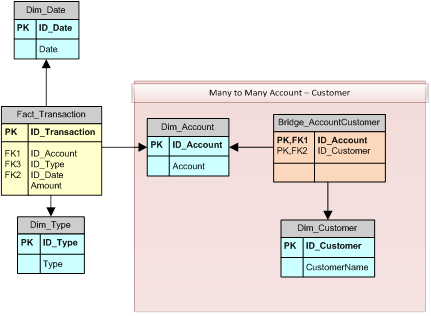If you want to obtain the total Amount for all the accounts of the selected group of customers, you have to split the operation in two steps: first, select the accounts, then apply the account filter to the Transaction table.

In order to filter the accounts, you would use the FILTER function returning only those accounts that have at least one related customer active in the current filter context. Because the filter context is automatically propagated following the one-to-many relationship, any selection applied to the Dim_Customer column propagates its effects to the Bridge_AccountCustomer table. The FILTER statement iterates the Dim_Account table; for each account the row context of Dim_Account is transformed into a filter context propagated to Bridge_AccountCustomer in the CALCULATE expression used in the second parameter of the FILTER call. At this point, counting the rows of Bridge_AccountCustomer will only return a number greater than 0 if the account in question belongs to one or more selected customers. This calculation can be implemented with the following formula, which can operate also with the first version of PowerPivot (released with SQL Server 2008 R2).

```AmountM2M :=

CALCULATE(
SUM( Fact_Transaction[Amount] ),
FILTER(
Dim_Account,
CALCULATE( COUNTROWS( Bridge_AccountCustomer ) ) > 0
)
)
```

The operation performed by using the filter requires an iteration — if you have one million accounts, one million CALCULATE( COUNTROWS( Bridge_AccountCustomer ) ) calls are required. With PowerPivot 2012 and Analysis Services 2012 Tabular it is possible to perform the same calculation more efficiently.

FILTER has to return a list of Dim_Account. We can obtain the same result by using the ID_Account column, which defines the link to both the Fact_Transaction and the Bridge_AccountCustomer tables. The new SUMMARIZE DAX function can be used to replace the previous FILTER. When you use SUMMARIZE, the first parameter is the table on which you want to perform the summarization. Then there are one or more parameters identifying which data columns need to be grouped. Finally you can add an optional pair of parameters defining a name and the expression to summarize for each group. In other words, the SUMMARIZE is semantically similar to a GROUP BY operation in SQL.

We can try to understand the consequent efficiency improvement by making a SQL comparison. The FILTER expression

```FILTER(
Dim_Account,
CALCULATE( COUNTROWS( Bridge_AccountCustomer ) ) > 0
)
<br>```

corresponds to the following SQL query:

```SELECT a.ID_Account
FROM   Dim_Account
WHERE  (SELECT COUNT(*)
FROM Bridge_AccountCustomer b
WHERE b.ID_Account = a.ID_Account
AND <filter context applies here>) > 0
WHERE <filter context applies here>
```

If we replace the previous FILTER with the following SUMMARIZE:

```SUMMARIZE(
Bridge_AccountCustomer,
Dim_Account[ID_Account]
)
```

This SUMMARIZE is semantically similar to the following SQL query:

```SELECT a.ID_Account
FROM   Bridge_AccountCustomer b
INNER JOIN Dim_Account a
WHERE <filter context applies here>
GROUP BY a.ID_Account
```

As you can see, SUMMARIZE is more concise and better expresses the type of operation. Of course, a smarter query optimizer might result in a similar query plan, but such optimization is hard to obtain from advanced RDBMS and it is not available in DAX engines today.

For this reason, it is important to use SUMMARIZE in order to get the best possible performance navigating M2M relationships in DAX. The following is the more optimized DAX measure based on SUMMARIZE:

```AmountM2M :=

CALCULATE(
SUM( Fact_Transaction[Amount] ),
SUMMARIZE(
Bridge_AccountCustomer,
Dim_Account[ID_Account]
)
)
```

Finally, there is another possible syntax available in DAX — it is harder to explain with a comparison with SQL but returns the same result as the SUMMARIZE technique handling many-to-many relationships. This approach has been described by Gerhard Brueckl and produces a very elegant calculation leveraging cross table filtering. You simply have to include in the CALCULATE filter parameters the bridge table and the intermediate dimension table involved in the many-to-many relationship. In this example, we are talking about the Bridge_AccountCustomer and the DimAccount tables.

```AmountM2M :=

CALCULATE(
SUM( Fact_Transaction[Amount] ),
Bridge_AccountCustomer
)
```

I ran tests on more complex models and this approach does perform similarly to the SUMMARIZE version, but it has the big advantage of requiring less knowledge of the underlying model. This makes it easier to be automatically generated by a client tool or simply by a developer who doesn’t want to worry about the field used to define a relationship.

You can find a more complete and deep discussion about many-to-many patterns in DAX and MDX in “The Many-to-Many Revolution 2.0” whitepaper.

FILTERReturns a table that has been filtered.

`FILTER ( <Table>, <FilterExpression> )`

CALCULATEContext transition

Evaluates an expression in a context modified by filters.

`CALCULATE ( <Expression> [, <Filter> [, <Filter> [, … ] ] ] )`

COUNTROWSCounts the number of rows in a table.

`COUNTROWS ( <Table> )`

SUMMARIZECreates a summary of the input table grouped by the specified columns.

`SUMMARIZE ( <Table> [, <GroupBy_ColumnName> [, [<Name>] [, [<Expression>] [, <GroupBy_ColumnName> [, [<Name>] [, [<Expression>] [, … ] ] ] ] ] ] ] )`